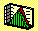4.11 CHAPTER 4: EXERCISES

In the following exercises an asterisk denotes greater difficulty.

1. Using the default data set (CAPM.DAT) in CAPM Tutor, the three-firm problem, start with the portfolio in which stocks 1 and 2 have zero weight, and stock 3 has weight equal to one. Now, change the weight of stock one continuously from zero to one, and look at the display change.

a) Explain what is being plotted, and how it is calculated.

b) Why does the curve "bend-back," i.e., why does the standard deviation first decrease and then increase?

c) Determine the portfolio weights at the point at which the curve bends, using the scroll bars in the Markowitz Diversification chapter of CAPM Tutor.

2a*) Describe the mathematical problem that allows you to solve for the portfolio weights in problem 1 c).

b*) Solve the mathematical problem described in the first part of this problem, and reconcile your answer with 1 c).

3. Using the data set INDUS.DAT, start with the portfolio in which ENERGY and FINANCE have zero weight, and PETRO has weight equal to 1. Now, change the weight of ENERGY continuously from 0 to 1, and look at the display change.

a) Explain what is being plotted, and how it is calculated.

b) Why does the curve "bend-back", i.e. why does the standard deviation first decrease and then increase?

Now introduce FINANCE into the portfolio by setting its weight to each of the following values:

0.1, 0.2, 0.3, 0.4, 0.5, 0.6, 0.7, 0.8.

To do this first change the Alpha (portfolio weight) for Finance to be 0.1 and then select Energy so that this industry is the portfolio weight that is varied.

c) Describe the effect in terms of attainable risk/return points of increasing the portfolio weight for finance.

d) If your target expected return is 0.00043 for the above set of portfolio weights for FINANCE, find the portfolio that provides the smallest portfolio standard deviation.

e) For the above set of portfolio weights for FINANCE, find the best expected return you can achieve for a portfolio standard deviation of 0.0075. Record the portfolio weights associated with this portfolio.

4. Using the file ENERGY.DAT, choose the equally weighted (i.e., naive diversification) option.

a) Compute the portfolio standard deviation and expected return for the naively diversified portfolio.

b) By altering the weights of any of the securities, find a portfolio that provides at least the same expected return but has lower portfolio standard deviation than the naive portfolio in a) above.

c) Find a portfolio that provides approximately the same portfolio standard deviation but higher expected return than the naive portfolio in a) above.

d) What basic principle is illustrated by your answers to parts b) and c) above?

5. Explain how a point on the efficient frontier is calculated. Using the default dataset (CAPM.DAT), calculate the optimal portfolio for a 15% return, and verify your answer using CAPM Tutor.

6. Using the dataset INDUS.DAT, construct a portfolio that has the smallest variance using Markowitz diversification.

a) Contrast the risk and return properties associated with the minimum-variance Markowitz portfolio with the naively diversified portfolio.

b) Does the minimum-variance Markowitz portfolio dominate the naive portfolio? (Provide support for your answer.)

7. The dataset ENGPET.DAT, contains the data sets ENERGY.DAT and PETRO.DAT pooled (i.e., this set contains 10 stocks).

a) Compute the minimum-variance Markowitz portfolio, and record it's risk/return properties.

b) Compute the naive portfolio, and record its risk/return properties.

c) Does the portfolio in a) dominate the portfolio in b)?

d) Using Markowitz diversification, state the relationship between portfolio size and portfolio risk? (Support your answer with an analysis of portfolios consisting of 1,4,7, and 10 stocks respectively.)

e) Using naive diversification state the relationship between portfolio size and portfolio risk? (Support your answer with an analysis of portfolios consisting of 1,4,7 and 10 stocks respectively.)

f) Compare and evaluate your findings in d) and e) above.

previous topic

next topic